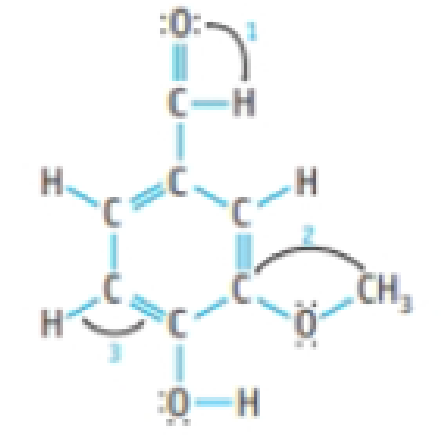Chapter 8, Problem 76GQ

Chapter
Section
Textbook Problem

Vanillin is the flavoring agent in vanilla extract and in vanilla ice cream. Its structure is shown here:(a) Give values for the three bond angles indicated. (b) Indicate the shortest carbon-oxygen bond in the molecule. (c) Indicate the most polar bond in the molecule.

(a)

Interpretation Introduction

Interpretation: The bond angle in the given compound should be determined.

Concept Introduction:

• In VSEPR, the geometry of the molecule is explained based on minimizing electrostatic repulsion between the molecules’ valence electrons around a central atom
• Bond angle is the angle between two bonds of a molecule and it is determined based on the electron-domain geometry.

[Bond angles: tetrahedral = 109.5o, trigonal planar = 120o, T-shape = 90o]

Electron geometry is the shape of a molecule predicted by considering both bond electron pairs and lone pair of electrons.

Molecular geometry is the shape of a molecule predicted by considering only bond pair of electrons.

Bond angle: It is defined as the angle between the orbital contacting bonding electron pair around the central atom in a molecule ion. Bond angle is expressed in degree.

Explanation

The compound is given below:

In bond angle 1 and 3, the geometry of central carbon atom is trigonal planar to minimize the electrostatic repulsion between the atom. Hence, the bond angle of O=CH and HC=

(b)

Interpretation Introduction

Interpretation:

The shorter carbon–oxygen bond in the molecule has to be determined.

Concept introduction:

Bond order: It is the measure of number of electron pairs shared between two atoms.

Bondorder=12(NumberofelectronsinbondoingMOs-NumberofelectronsinantibondingMOs)

Bond length is inversely proportional to the bond order.

(c)

Interpretation Introduction

Interpretation: The most polar bond in the given compound should be determined.

Concept Introduction:

Polarity: It is a well separation of electric charge leading to a molecule or chemical compounds having an electrical dipole moment. Generally the polar molecules must contain polar bonds due to a different in electronegative between the bonded atoms.

Still sussing out bartleby?

Check out a sample textbook solution.

See a sample solution

The Solution to Your Study Problems

Bartleby provides explanations to thousands of textbook problems written by our experts, many with advanced degrees!

Get Started

Find more solutions based on key concepts